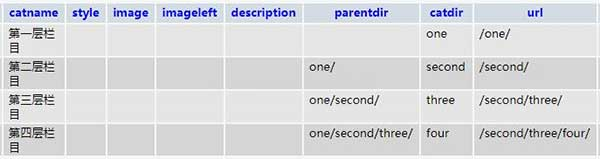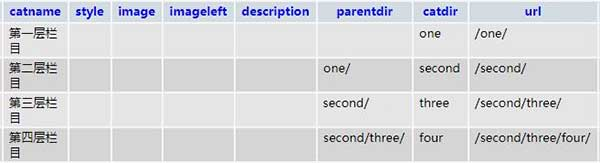15621857753

# PHPCMSV9栏目设置是否生成到根目录后路径错误Bug如何修复\$arrparentid = explode(',', \$arrparentid);
\$arrcatdir = array();
foreach(\$arrparentid as \$id) {
if(\$id==0) continue;
\$arrcatdir[] = \$this->categorys[\$id]['catdir'];
}
return implode('/', \$arrcatdir).'/';

\$arrparentid = explode(',', \$arrparentid);
\$arrcatdir = array();
foreach(\$arrparentid as \$id) {
if(\$id==0) continue;
\$arrcatdir[] = \$this->categorys[\$id]['catdir'];
}
\$parentdirs = implode('/', \$arrcatdir).'/';
\$temp_categorys = \$this->db->select(array('siteid'=>\$this->siteid,'module'=>'content'), '*', '', 'listorder ASC, catid ASC', '', 'catid');
foreach(\$arrparentid as \$id) {
\$temp_r = array();
\$temp_r = \$temp_categorys[\$id];
\$temp_setting = string2array(\$temp_r['setting']);
if(\$temp_setting['create_to_html_root']){
\$temp_parentid = \$temp_categorys[\$id]['parentid'];
\$parent_r = \$temp_categorys[\$temp_parentid];
\$parentdirs = str_replace(\$parent_r['catdir'].'/', '', \$parentdirs);
}
}
return \$parentdirs;